Background
We will work with the standard triangular representation of a regression specification and assume the existence of a single cointegrating vector as in Hansen (1992). Consider a panel structure for the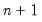dimensional time series vector process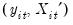, with cointegrating equation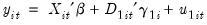(56.1)
for cross-sections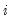and periods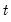, where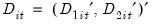are deterministic trend regressors and the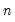stochastic regressors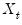are governed by the system of equations: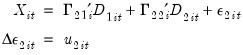(56.2)
The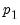-vector of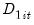regressors enter into both the cointegrating equation and the regressors equations, while the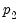-vector of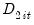are deterministic trend regressors which are included in the regressors equations but excluded from the cointegrating equation (see “Cointegrating Regression” for further discussion).
It is worth mentioning that most authors have focused attention on the leading case in which the deterministic trend terms in the panel cointegrating equation consist only of cross-section dummy variables: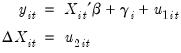(56.3)
Notice that the cointegrating relationship between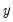and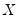is assumed to be homogeneous across cross-sections, and that the specification allows for cross-section specific deterministic effects.
Following Phillips and Moon (1999), we define the long run covariance matrices for the errors in cross-section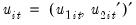are strictly stationary and ergodic with zero mean, contemporaneous covariance matrix, one-sided long-run covariance matrix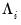, and long-run covariance matrix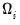, each of which we partition conformably with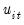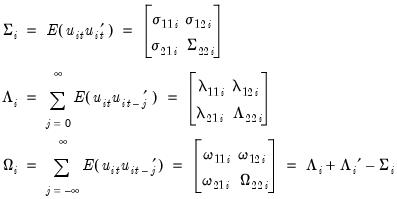(56.4)
and define what Phillips and Moon term the long-run average covariance matrices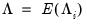, and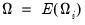.
Lastly, and perhaps most importantly, we assume that there is independence in the errors across cross-sections.
Given this basic structure, we may define panel estimators of the cointegrating relationship coefficient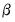using extensions of single-equation FMOLS and DOLS methods. There are different variants for each of the estimators depending on the assumptions that one wishes to make about the long-run covariances and how one wishes to use the panel structure of the data.
We begin by describing how to estimate panel FMOLS and DOLS models in EViews. We then discuss the views and procedures associated with the panel equation and offer a simple example. Lastly, we provide technical details on the estimation methods.